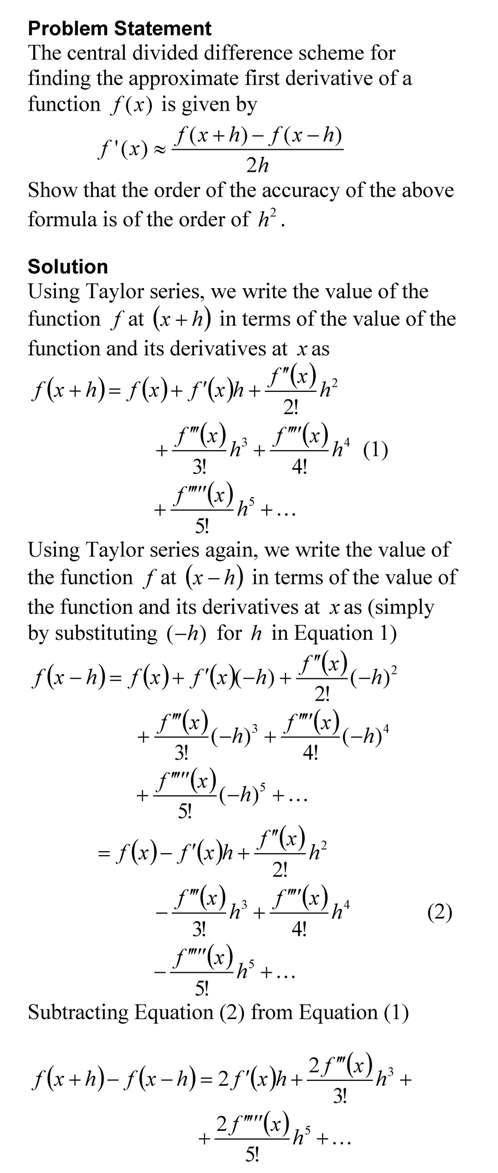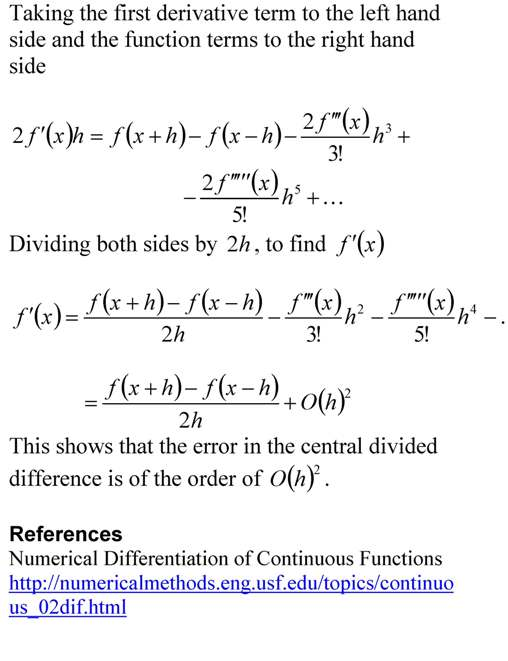## An FE Exam Math Problem in Differential Calculus

“The Fundamentals of Engineering (FE) exam is generally the first step in the process of becoming a professional licensed engineer (P.E.). It is designed for recent graduates and students who are close to finishing an undergraduate engineering degree from an EAC/ABET-accredited program” – FE Exam NCEES

For most engineering majors, mathematics is a required part of the examination. Here is a question from calculus.This post is brought to you by

• Holistic Numerical Methods Open Course Ware:
• the textbooks on
• the Massive Open Online Course (MOOCs) available at

## Differentiating a Discrete Function with Equidistant Points

Many students ask me how do I do this or that in MATLAB.  This is a new addition to the “How do I do that in MATLAB”  series.

In this blog, I show you how to find the first derivative of a discrete function y(x).  We are assuming that the x values are eqidistant and the data is sorted in ascending or descending order by the x values.  The latter requirement can be relaxed easily in programs such as MATLAB where one can use the sortrows command to put the data in the required order.

To keep the accuracy of all calculated first derivatives to be the same, we use the following formulas:

For the first data point, we use the forward divided difference formula
f ‘(x) =(-f(x+2h) + 4 f(x+h) -3 f(x))/(2h)+order(h^2)

For the interior points, we use the central divided difference formula
f ‘(x) =(f(x+h) -f(x-h))/(2h)+order(h^2)

For the last data point, we use the backward divided difference formula
f ‘(x) =(f(x-2h) – 4 f(x-h) +3 f(x))/(2h)+order(h^2)

Here are the links for the program.
The mfile is here
The published version of the mfile is here

%% HOW DO I DO THAT IN MATLAB SERIES?
% In this series, I am answering questions that students have asked
% me about MATLAB.  Most of the questions relate to a mathematical
% procedure.

%% TOPIC
% How do I find the first derivative of a discrete function y(x) if the
% x values are equidistant.
%% SUMMARY
% Language : Matlab 2010a;
% Authors : Autar Kaw and Sri Garapati;
% Mfile available at
% http://numericalmethods.eng.usf.edu/blog/discrete_diff_equidistant_blog.m
% Last Revised : January 17, 2012;
% Abstract: This program shows you how to differentiate discrete data if
% the x values are equally spaced
clc
clear all

%% INTRODUCTION

disp(‘ABSTRACT’)
disp(‘   This program shows you how to differentiate discrete data if’)
disp(‘   the x values are equally spaced ‘)

disp(‘ ‘)
disp(‘AUTHOR’)
disp(‘   Autar Kaw and Sri Garapati of https://autarkaw.wordpress.com’)
disp(‘ ‘)
disp(‘MFILE SOURCE’)
disp(‘   http://numericalmethods.eng.usf.edu/blog/discrete_diff_equidistant_blog.m’)
disp(‘ ‘)
disp(‘LAST REVISED’)
disp(‘   January 17, 2012’)
disp(‘ ‘)

%% INPUTS

% Inputs assuming
%    that three or more points are given
%    all x values are equidistant
%    x values are in ascending or descending order.
x=[2.3   3.4   4.5    5.6   6.7   7.8];
y=[4.6   7.9   13.0   12.3  3.2   1.9];
%% DISPLAYING INPUTS
disp(‘  ‘)
disp(‘INPUTS’)
% Creating a matrix to print input data as a table
data=[x;y]’;
disp(‘   X Data     Y Data’)
% Printing the input data as a table
disp(data)

%% THE CODE

% n returns the number of data points
n=length(x);
% delta is the distance between consecutive x values
delta=x(2)-x(1);

% “dy” is an array which stores the value of the derivative at each x-value

% finding the derivative from the 2nd order accurate forward divided
% difference formula at the first data point
dy(1)=(-y(3)+4*y(2)-3*y(1))/(2*delta);

% finding the derivative from the 2nd order accurate central divided
% difference formula at the second to (n-1)th data point
for i=2:1:n-1
dy(i)=(y(i+1)-y(i-1))/(2*delta);
end

% finding the derivative from the 2nd order accurate backward divided
% difference formula at the first data point
dy(n)=(y(n-2)-4*y(n-1)+3*y(n))/(2*delta);

% creating a matrix with input data and calculated derivative value at
% each data point for printing as a table
xdy=[x’ y’ dy’];

%% DISPLAYING OUTPUTS
disp(‘  ‘)
disp(‘OUTPUTS’)
disp(‘     XData   YData  Derivative’)
% printing the input data points and calculated derivative values(Outputs)
disp(xdy)
_________________________________________________

This post is brought to you by Holistic Numerical Methods: Numerical Methods for the STEM undergraduate at http://numericalmethods.eng.usf.edu, the textbook on Numerical Methods with Applications available from the lulu storefront, the textbook on Introduction to Programming Concepts Using MATLAB, and the YouTube video lectures available at http://numericalmethods.eng.usf.edu/videos.  Subscribe to the blog via a reader or email to stay updated with this blog. Let the information follow you.

## Order of accuracy of central divided difference scheme for first derivative of a function of one variableThis post is brought to you by
Holistic Numerical Methods: Numerical Methods for the STEM undergraduate at http://numericalmethods.eng.usf.edu, the textbook on Numerical Methods with Applications available from the lulu storefront, the textbook on Introduction to Programming Concepts Using MATLAB, and the YouTube video lectures available at http://numericalmethods.eng.usf.edu/videos.  Subscribe to the blog via a reader or email to stay updated with this blog. Let the information follow you.

## How do I differentiate in MATLAB?

Many students ask me how do I do this or that in MATLAB.  So I thought why not have a small series of my next few blogs do that.  In this blog, I show you how to differentiate a function.

The MATLAB program link is here.

The HTML version of the MATLAB program is here.

___________________________________________

%% HOW DO I DO THAT IN MATLAB SERIES?
% In this series, I am answering questions that students have asked
% me about MATLAB.  Most of the questions relate to a mathematical
% procedure.

%% TOPIC
% How do I differentiate a function?

%% SUMMARY
% Language : Matlab 2008a
% Authors : Autar Kaw
% Mfile available at
% http://nm.mathforcollege.com/blog/differentiation.m
% Last Revised : March 21, 2009
% Abstract: This program shows you how to differentiate a given function

%% INTRODUCTION
clc
clear all
disp(‘ABSTRACT’)
disp(‘   This program shows you how to differentiate’)
disp(‘   a given function and then find its value’)
disp(‘   at a given point’)
disp(‘ ‘)
disp(‘AUTHOR’)
disp(‘   Autar K Kaw of https://autarkaw.wordpress.com&#8217;)
disp(‘ ‘)
disp(‘MFILE SOURCE’)
disp(‘   http://nm.mathforcollege.com/blog/differentiation.m&#8217;)
disp(‘ ‘)
disp(‘LAST REVISED’)
disp(‘   March 21, 2009’)
disp(‘ ‘)

%% INPUTS

% Differentiate 7 exp(3*x) once and find the value of the
% first derivative at x=0.5
% Define x as a symbol
syms x
% Defining the function to be differentiated
y=7*exp(3*x);
% Defining the point where you want to find the derivative
xx=0.5;

%% DISPLAYING INPUTS
disp(‘INPUTS’)
func=[‘  The function is to be differentiated is ‘ char(y)];
disp(func)
fprintf(‘  Value of x where you want to find the derivative, x= %g’,xx)
disp(‘  ‘)
disp(‘  ‘)

%% THE CODE
% Finding the derivative using the diff command
% Argument 1 is the function to be differentiated
% Argument 2 is the variable with respect to which the
%    function is to be differentiated – the independent variable
% Argument 3 is the order of derivative
dydx=diff(y,x,1);
% subs command substitues the value of x
dydx_val=subs(dydx,x,xx);
%% DISPLAYING OUTPUTS
disp(‘OUTPUTS’)
derivative_func=[‘  The derivative of function ‘ char(y) ‘ is ‘ char(dydx)];
disp(derivative_func)
fprintf(‘  Value of dydx at x=%g is =%g’,xx,dydx_val)
disp(‘  ‘)

______________________________________________________________________

This post is brought to you by Holistic Numerical Methods: Numerical Methods for the STEM undergraduate at http://nm.mathforcollege.com, the textbook on Numerical Methods with Applications available from the lulu storefront, and the YouTube video lectures available at http://nm.mathforcollege.com/videos

## Data for aluminum cylinder in iced water experiment

A colleague asked me what if he did not have time or resources to do the experiments that have been developed at University of South Florida (USF) for numerical methods. He asked if I could share the data taken at USF.

Why not – here is the data for the experiment where an aluminum cylinder is placed in iced water. This link also has the exercises that the students were asked to do.

The temperature vs time data is as follows: (0,23.3), (5,16.3), (10,13), (15,11.8), (20,11), (25,10.7), (30,9.6), (35,8.9), (40,8.4). Time is in seconds and temperature in Celcius. Other data needed is

Ambient temperature of iced water = 1.1oC

Diameter of cylinder = 44.57 mm

Length of cylinder = 105.47 mm

Density of aluminum = 2700 kg/m3

Specific heat of aluminum = 901 J/(kg-oC)

Thermal conductivity of aluminum = 240 W/(m-K)

Table 1. Coefficient of thermal expansion vs. temperature for aluminum (Data taken from http://www.llnl.gov/tid/lof/documents/pdf/322526.pdf by using mid values of temperatures at which CTE is reported)

 Temperature (oC) Coefficient of thermal expansion (μm/m/oC) -10 58 12.5 59 37.5 60 62.5 62 87.5 66 112.5 71

This post is brought to you by Holistic Numerical Methods: Numerical Methods for the STEM undergraduate at http://numericalmethods.eng.usf.edu